## Topic: Statistics

Free Printable Worksheets on Statistics

## General Statistics Worksheet – A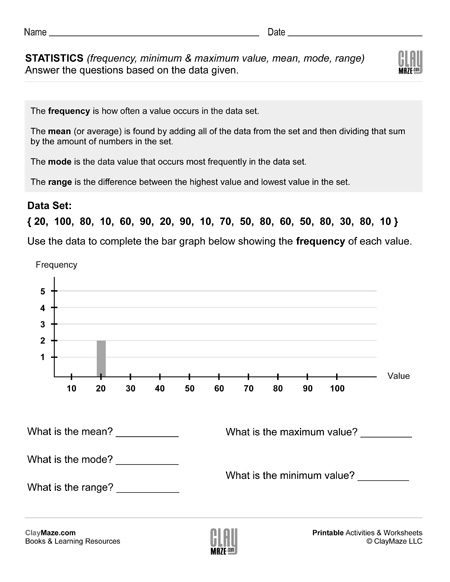This worksheet has a few statistics terms defined at the top. Following that is a data set and a graph to fill out using the data given. Below that are questions about the data which use the...

## General Statistics Worksheet – BThere are statistics terms defined at the top of this worksheet followed by a data set and a graph to be filled out using the data set. Then the student asked questions about the data using the...

## General Statistics Worksheet – C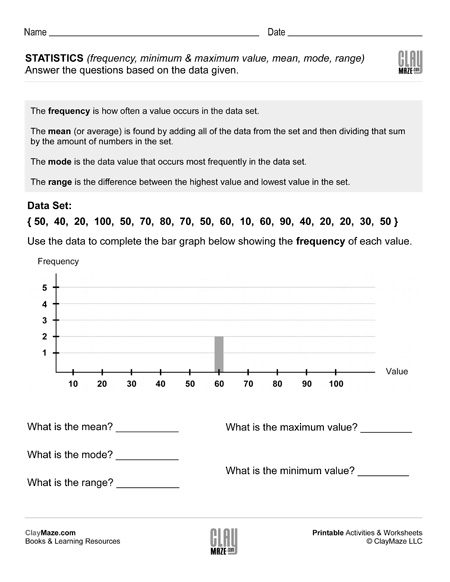The worksheet has statistics terms defined at the top for reference. Below that is a set of data values. Using these values, the student is asked to complete the graph. Following the graphs are...

## General Statistics Worksheet – DThis worksheet gives a data set that should be used to complete the graph and answer the questions. The student can refer to the statistics terms defined at the top of the worksheet to answer the...

## Statistics: Minimum, Maximum, Mean, Median, Mode, Range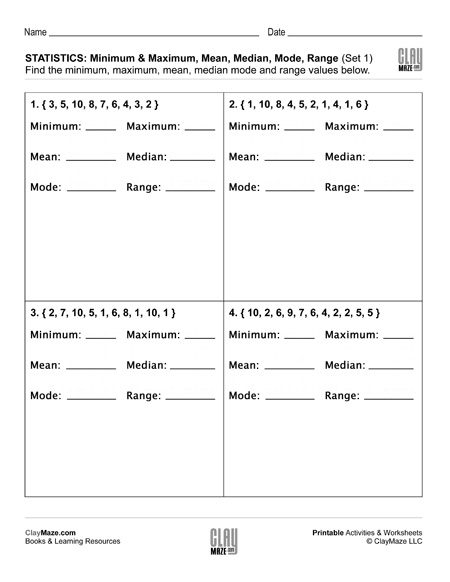Each of the statistics worksheets in this set contains 4 sets of data. The student is asked to find the minimum, maximum, mean, median, mode and range for each data set. These are recommended...

## Basic Statistics: Probability I (Set 1)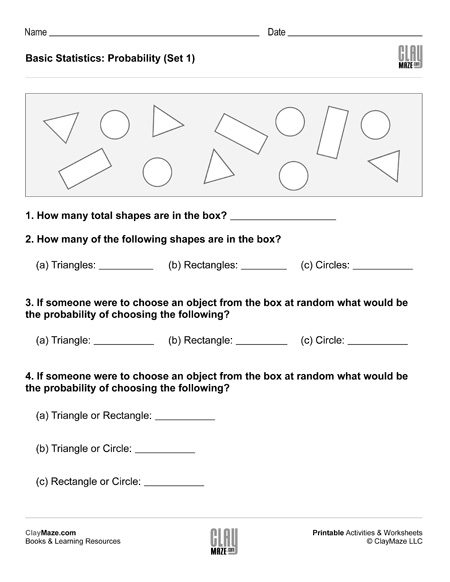This statistics worksheet is on basic probability. There are a number of different shapes in the box and the student is asked to find the probabilities of selecting the different shapes at random....

## Basic Statistics: Probability I (Set 2)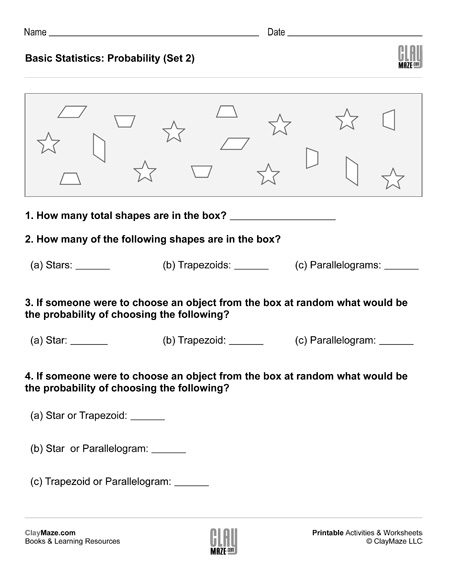This free worksheet covers basic probability. The student is asked several questions about the number of shapes in the box. The student can then use those values to find the probabilities of...

## Basic Statistics: Probability I (Set 3)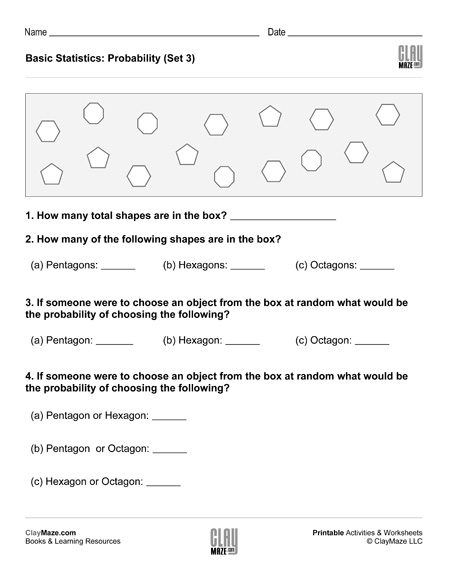This is a basic probability worksheet. The questions are based on the number of shapes in the box and the student is asked to find the probabilities of randomly choosing the different shapes....

## Basic Statistics: Probability I (Set 4)Worksheet on basic probability – The student is asked several questions about the number of shapes in the box and to find the probabilities of selecting different shapes at random....